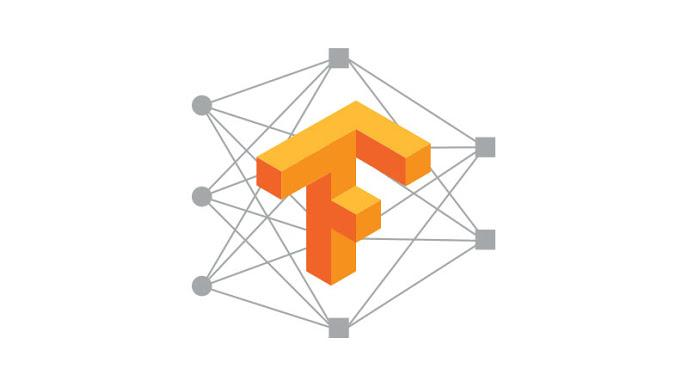• 一个完整的、循序渐进的学习TensorFlow中RNN实现的方法。这个学习路径的曲线较为平缓，应该可以减少不少学习精力，帮助大家少走弯路。
• 一些可能会踩的坑
• TensorFlow源码分析
• 一个Char RNN实现示例，可以用来写诗，生成歌词，甚至可以用来写网络小说！（项目地址：hzy46/Char-RNN-TensorFlow

## 一、学习单步的RNN：RNNCell

• state_size
• output_size

``````import tensorflow as tf
import numpy as np

cell = tf.nn.rnn_cell.BasicRNNCell(num_units=128) # state_size = 128
print(cell.state_size) # 128

inputs = tf.placeholder(np.float32, shape=(32, 100)) # 32 是 batch_size
h0 = cell.zero_state(32, np.float32) # 通过zero_state得到一个全0的初始状态，形状为(batch_size, state_size)
output, h1 = cell.call(inputs, h0) #调用call函数

print(h1.shape) # (32, 128)``````

``````import tensorflow as tf
import numpy as np
lstm_cell = tf.nn.rnn_cell.BasicLSTMCell(num_units=128)
inputs = tf.placeholder(np.float32, shape=(32, 100)) # 32 是 batch_size
h0 = lstm_cell.zero_state(32, np.float32) # 通过zero_state得到一个全0的初始状态
output, h1 = lstm_cell.call(inputs, h0)

print(h1.h)  # shape=(32, 128)
print(h1.c)  # shape=(32, 128)``````

## 二、学习如何一次执行多步：tf.nn.dynamic_rnn

``````# inputs: shape = (batch_size, time_steps, input_size)
# cell: RNNCell
# initial_state: shape = (batch_size, cell.state_size)。初始状态。一般可以取零矩阵
outputs, state = tf.nn.dynamic_rnn(cell, inputs, initial_state=initial_state)``````

## 三、学习如何堆叠RNNCell：MultiRNNCell

``````import tensorflow as tf
import numpy as np

# 每调用一次这个函数就返回一个BasicRNNCell
def get_a_cell():
return tf.nn.rnn_cell.BasicRNNCell(num_units=128)
# 用tf.nn.rnn_cell MultiRNNCell创建3层RNN
cell = tf.nn.rnn_cell.MultiRNNCell([get_a_cell() for _ in range(3)]) # 3层RNN
# 得到的cell实际也是RNNCell的子类
# 它的state_size是(128, 128, 128)
# (128, 128, 128)并不是128x128x128的意思
# 而是表示共有3个隐层状态，每个隐层状态的大小为128
print(cell.state_size) # (128, 128, 128)
# 使用对应的call函数
inputs = tf.placeholder(np.float32, shape=(32, 100)) # 32 是 batch_size
h0 = cell.zero_state(32, np.float32) # 通过zero_state得到一个全0的初始状态
output, h1 = cell.call(inputs, h0)
print(h1) # tuple中含有3个32x128的向量``````

## 四、可能遇到的坑1：Output说明

``````def call(self, inputs, state):
"""Most basic RNN: output = new_state = act(W * input + U * state + B)."""
output = self._activation(_linear([inputs, state], self._num_units, True))
return output, output``````

``````new_c = (
c * sigmoid(f + self._forget_bias) + sigmoid(i) * self._activation(j))
new_h = self._activation(new_c) * sigmoid(o)

if self._state_is_tuple:
new_state = LSTMStateTuple(new_c, new_h)
else:
new_state = array_ops.concat([new_c, new_h], 1)
return new_h, new_state``````

## 五、可能遇到的坑2：因版本原因引起的错误

``````# 每调用一次这个函数就返回一个BasicRNNCell
def get_a_cell():
return tf.nn.rnn_cell.BasicRNNCell(num_units=128)
# 用tf.nn.rnn_cell MultiRNNCell创建3层RNN
cell = tf.nn.rnn_cell.MultiRNNCell([get_a_cell() for _ in range(3)]) # 3层RNN``````

``````one_cell =  tf.nn.rnn_cell.BasicRNNCell(num_units=128)
cell = tf.nn.rnn_cell.MultiRNNCell([one_cell] * 3) # 3层RNN``````

## 六、一个练手项目：Char RNN

Char RNN的实现已经有很多了，可以自己去Github上面找，我这里也做了一个实现，供大家参考。项目地址为：hzy46/Char-RNN-TensorFlow。代码的部分实现来自于这篇专栏，在此感谢 @天雨粟

``````何人无不见，此地自何如。

``````static int page_cpus(struct flags *str)
{
int rc;
struct rq *do_init;
};

/*
* Core_trace_periods the time in is is that supsed,
*/
#endif

/*
* Intendifint to state anded.
*/
int print_init(struct priority *rt)
{       /* Comment sighind if see task so and the sections */
console(string, &can);
}``````

``````LAUNCE:
The formity so mistalied on his, thou hast she was
to her hears, what we shall be that say a soun man
Would the lord and all a fouls and too, the say,
That we destent and here with my peace.

PALINA:
Why, are the must thou art breath or thy saming,
I have sate it him with too to have me of
I the camples.``````

``````闻言，萧炎一怔，旋即目光转向一旁的那名灰袍青年，然后目光在那位老者身上扫过，那里，一个巨大的石台上，有着一个巨大的巨坑，一些黑色光柱，正在从中，一道巨大的黑色巨蟒，一股极度恐怖的气息，从天空上暴射而出 ，然后在其中一些一道道目光中，闪电般的出现在了那些人影，在那种灵魂之中，却是有着许些强者的感觉，在他们面前，那一道道身影，却是如同一道黑影一般，在那一道道目光中，在这片天地间，在那巨大的空间中，弥漫而开……

“这是一位斗尊阶别，不过不管你，也不可能会出手，那些家伙，可以为了这里，这里也是能够有着一些异常，而且他，也是不能将其他人给你的灵魂，所以，这些事，我也是不可能将这一个人的强者给吞天蟒，这般一次，我们的实力，便是能够将之击杀……”

“这里的人，也是能够与魂殿强者抗衡。”

“嗤！”``````